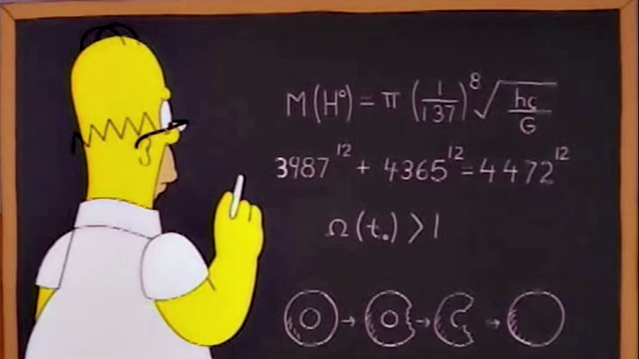# Maths on a Mug #9

Posted on

Fermat’s Last Theorem is a celebrated result in mathematics, simply it says that:

There are no three positive integers $$a,\,b,\,c$$ such that $$a^n+b^n=c^n$$ for any value of $$n>2$$.

For the value $$n=2$$ this is the well known Pythagoras theorem. A simple theorem to state, but which was fiendishly hard to prove. It was first stated by Fermat in 1637 and took until May of 1995 to be finally proved by Sir Andrew Wiles. However there is a fun ‘near-miss’ solution which was shown in the Simpsons, and is on the mug this morning. If you type this solution into your handy pocket calculator you will think you’ve found a counterexample! However the numbers are so large ($$4472^{12}=63976656348486725806862358322168575784124416$$) that your calculator doesn’t actually work out the whole values and there is a ’rounding error’. A handy video to explain this is here.### Sean

This site uses Akismet to reduce spam. Learn how your comment data is processed.

Related Posts